# RD Sharma Solutions Class 7 Chapter 2 Fraction

Read RD Sharma Solutions Class 7 Chapter 2 Fraction below, students should study RD Sharma class 7 Mathematics available on Studiestoday.com with solved questions and answers. These chapter wise answers for class 7 Mathematics have been prepared by teacher of Grade 7. These RD Sharma class 7 Solutions have been designed as per the latest NCERT syllabus for class 7 and if practiced thoroughly can help you to score good marks in standard 7 Mathematics class tests and examinations

Exercise 2.1

Question 1: Compare the following fractions by using the symbol > or < or =:
(i) (7/9) and  (8/13)
(ii) (11/9)and (5/9)
(iii) (37/41) and (19/30)
(iv) (17/15) and (119/105)

Solution 1: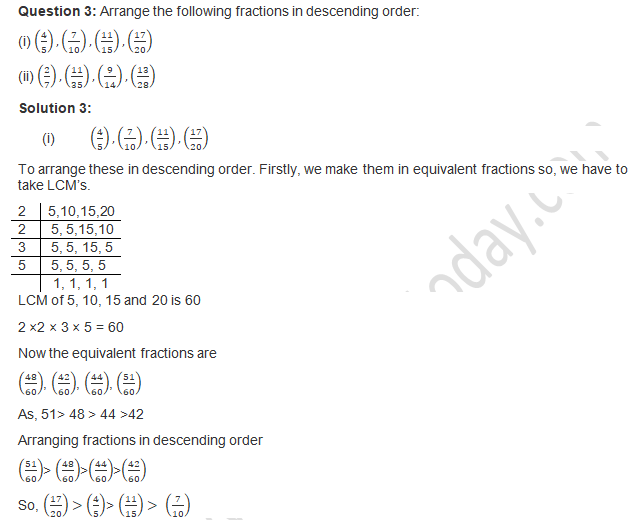Question 4: Write five equivalent fraction of (3/5).

Solution:

(3/5)
By multiplying the same number in numerator and denominator so that it keeps the same value by this,
Then we can get the equivalent fractions.
=((3 ×2  )/(5 ×2))  =(6/10)
((3 ×3  )/(5 ×3))=(9/15)
((3 ×4  )/(5 ×4))  = (12/20)
((3 ×5 )/(5 ×5))  = (15/25)
((3 ×6 )/(5 ×6))= (18/30)
So, the Equivalent fractions are
=(6/10),(9/15),(12/20)  ,(15/25),(18/30)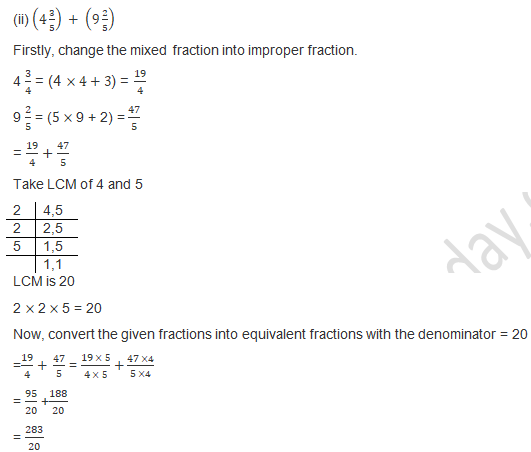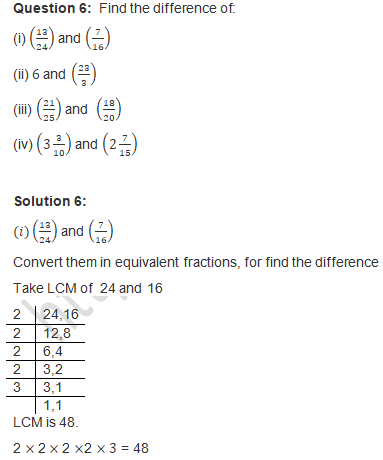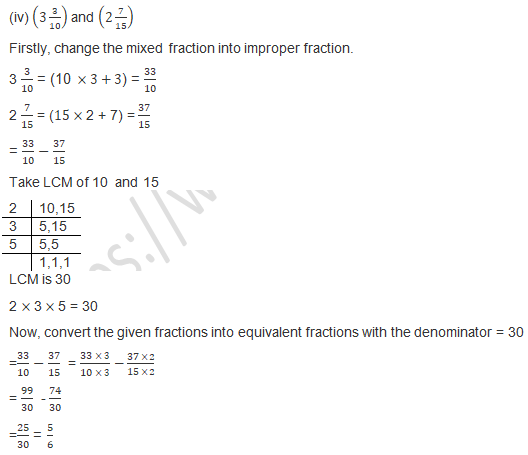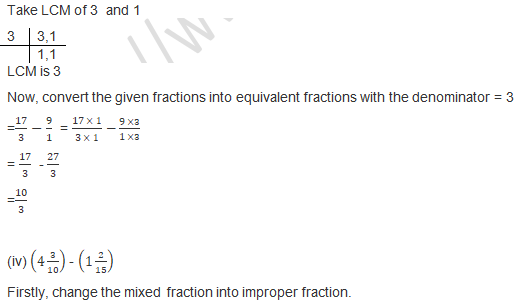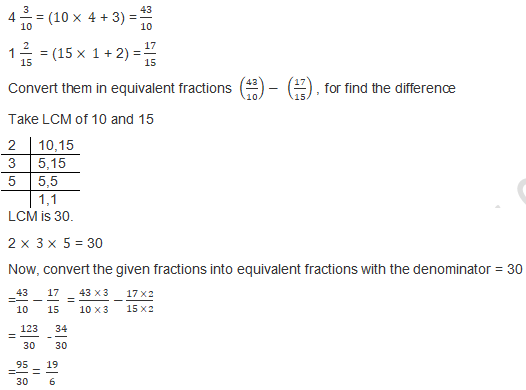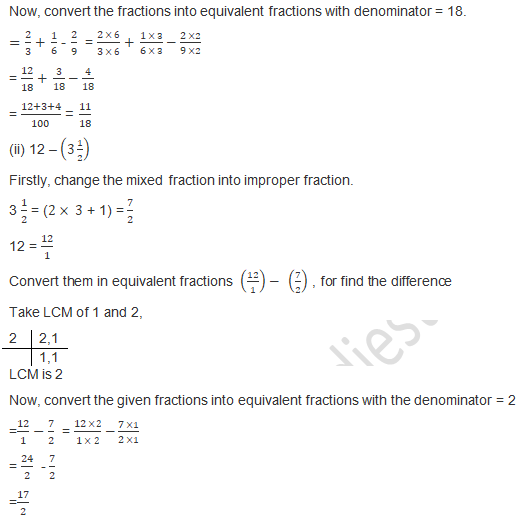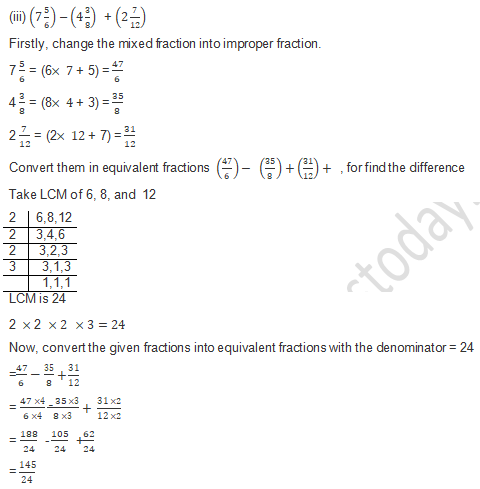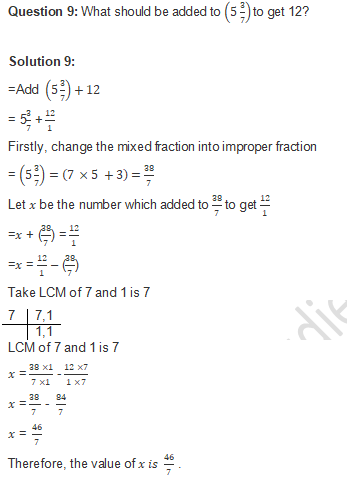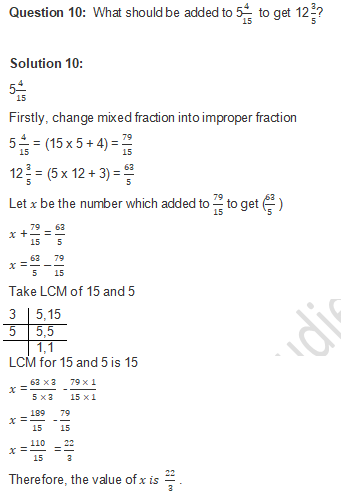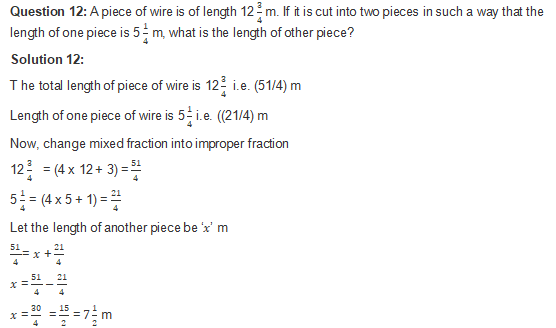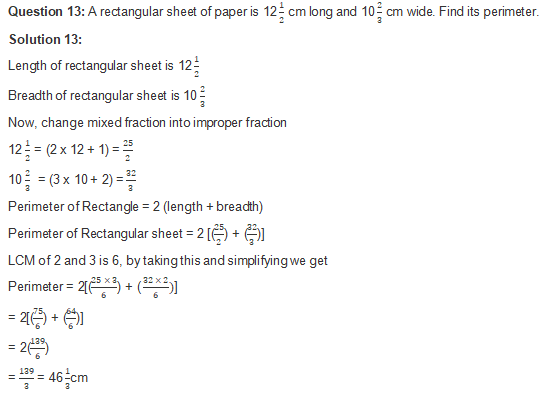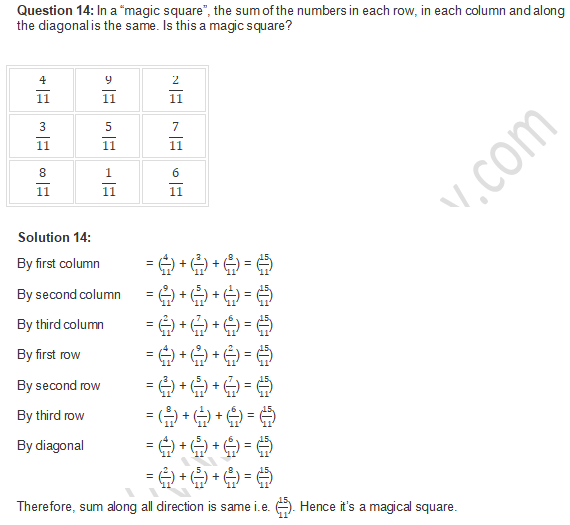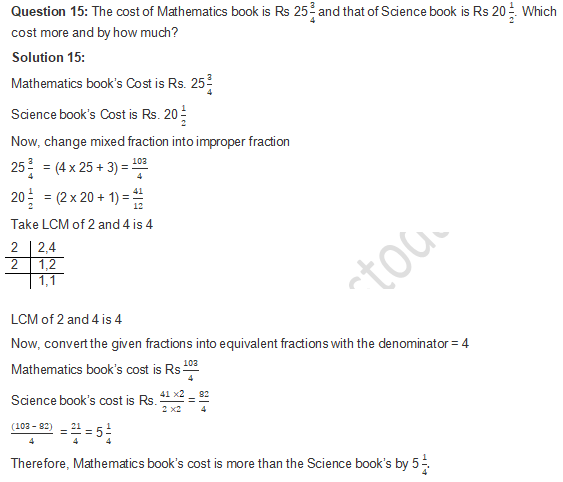Question 16. Provide the number in box [ ] and also give its simplest form in each of the following:
(i) 2/3 × [ ] = 10/30
(ii) 3/5 × [ ] =   24/75

Solution 16:

(i) 2/3 × [5/10] = 10/30
(ii) 3/5 × [ 8/15] =   24/75

Exercise 2.2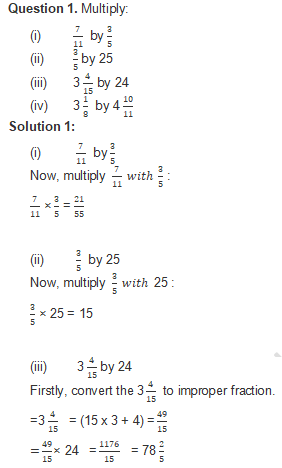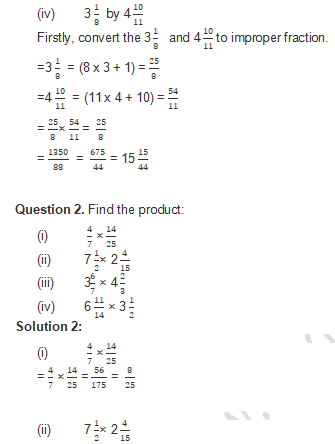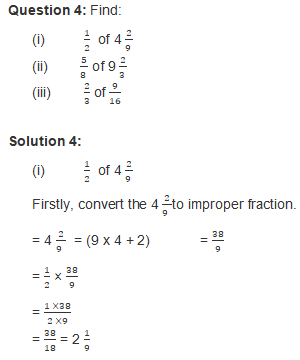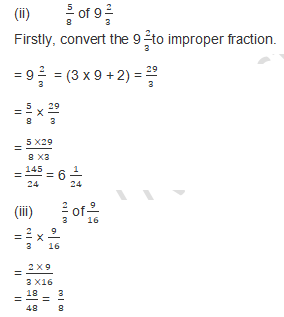Question 5. Which is greater?  1/2 of 6/7 or 2/3 of   3/7

Solution 5:

According to the question 1/2 of 6/7
= 1/2 × 6/7
= (1 ×6 )/(2 × 7)
= 6/14
Also given that 2/3 of   3/7
= 2/3 ×   3/7
= (2 ×3 )/(3 ×7 )  = 6/21
When two fractions are compared, if the numerators of both fractions are the same, the denominator with a higher value indicates that the fraction has a lower value.
Therefore 6/14 is greater.
Therefore,  1/2 of 6/7 is greater.

Question 6: Find:
(i) 7/11 of Rs 330
(ii) 5/9 of 108 meters
(iii) 3/7 of 42 liters
(iv) 1/12 of an hour
(v) 5/6 of an year
(vi) 3/20 of a kg
(vii) 7/20 of a liter
(viii) 5/6 of a day
(ix) 2/7  of a week

Solution 6:

(i) 7/11 of Rs 330
= 7/11 × 330 (330 is dividing by 11)
= 7 × 30
= 210 Rs.
So, the 7/11 of Rs 330 is Rs 210

(ii) 5/9 of 108 meters
= 5/9 × 108 (108 id dividing by 9)
= 5 × 12
= 60
So, the 5/9 of 108 meters is 60 meters

(iii) 3/7 of 42 liters
= 3/7 × 42 (42 is divided by 7)
= 3 × 6
= 18
So, the 3/7 of 42 liters is 18 liters

(iv) 1/12 of an hour
An hour = 60 minutes
= 1/12 × 60 (60 is divided by 12)
= 1 × 5
= 5
So, the 1/12 of an hour is 5 minutes

(v) 5/6 of an year
1 year = 12 months
= 5/6 × 12 (12 is divided by 6)
= 5 × 2
= 10
So, the 5/6 of an year is 10 months

(vi) 3/20 of a kg
1 kg = 1000 grams
= 3/20 × 1000 (1000 is dividing by 20)
= 3 × 50
= 150
So, the 3/20 of a kg is 150 grams.

(vii) 7/20 of a liter
1 liter = 1000 ml
= 7/20 × 1000 (1000 is dividing by 20)
= 7 × 50
= 350
So, the 7/20of a liter is 350ml.

(viii) 5/6 of a day
1 day = 24 hours
= 5/6 × 24 (24 is dividing by 6)
= 5 × 4
= 20
So, the 5/6 of a day is 20 hours

(ix) 2/7  of a week
1 week = 7 days
= 2/7 × 7(7 is dividing by 7)
= 2 × 1
= 2
So, the 2/7 of a week is 2 days

Question 7: Shikha plants 5 saplings in a row in her garden. The distance between two adjacent saplings is 3/4  m. Find the distance between the first and the last sapling.

Solution 7:

The distance between two adjacent saplings is 3/4 m
4 adjacent saplings are there for 5 saplings
So, the distance between the starting and the ending sapling is
= 3/4× 4 (4 is divided by 4)
= 3
Hence, The distance between from starting and ending sapling is 3 m.

Question 8: Ravish reads 1/3  part of a book in 1 hour. How much part of the book will he read in 2 1/5  hours?

Solution 8:

Ravish reads 1/3  part of the book in 1 hour
Now, calculate how much part he will read in 2 1/5  hours
Firstly, convert the 2 1/5  to improper fraction.
= 2 1/= (5 x 2 + 1) = 11/5
Let x = the full part of book
1 hour = 1/3 × x = 1/3 x
Further part of the book, he will read in
= 11/5  × (1 )/3=x
=(11 ×1)/( 5 ×3)  =x
= 11/15  part of the book

Question 9: Lipika reads a book for 1 3/4  3/4 hours every day. She reads the entire book in 6 days. How many hours in all were required by her to read the book?

Solution 9:

Lipika taken time to read a book per day = 1 3/4
Covert mixed fraction into improper fraction
=1 3/=( 4 ×1 +3) =  7/4
Lipika taken time to read a book in 6 days
= 7/4  × 6 (7 × 6 = 42)
=  42/4
= 10 ½ hours are consumed by Lipika to read 6 books.

Question 10: Find the area of a rectangular park which is 41 2/3 m along and 18 3/5 m broad.

Solution 10:

Given length of rectangular park is = 41 2/3
Covert mixed fraction into improper fraction
=41 2/3=( 3 ×41 +2) =  125/3
Rectangular park’s Breadth = 18 3/5 =  93/5
Covert mixed fraction into improper fraction
=18 3/5=( 5 ×18 +3) =  93/5
Rectangular park’s Area = length × breadth
= 125/3  × 93/5
= (125× 93)/(3 × 5)
= 11625/15
= 775 m2  is the area of the rectangular part.

Question 11: If milk is available at Rs 17 3/4  per liter, find the cost of 7 2/liters of milk.

Solution 11:

Given the cost of milk per liter is = 17 3/4
Covert mixed fraction into improper fraction
=17 3/4  =( 4 ×17+3) =   Rs. 71/4
And the cost of 7 2/5
Covert mixed fraction into improper fraction
=7 2/5  =( 5 ×7+2) =   Rs. 37/5
= 37/5  × 71/4
= (37 × 71)/(4 ×5 )
=  2627/20 = 131 7/4
Hence, The cost of 7 2/5 liters of milk is Rs. 131 7/4

Question 12: Sharada can walk 8 1/3  km in one hour. How much distance will she cover in 2 2/5  hours?

Solution 12:

Sharada covered Distance in one hour = 8 1/3
Covert mixed fraction into improper fraction
=8 1/3 =( 3 ×8+1) =   Rs. 25/3   km
Distance covered by her in 2 2/5   hours =  12/5
= 25/3  × 12/5
= (25 × 12)/( 3 × 5)
= 300/15
= 20 km
She covers distance in 2 2/5  hours in 20 km.

Question 13: A sugar bag contains 30kg of sugar. After consuming 2/3   of it, how much sugar is left in the bag?

Solution 13:

A sugar bag contains 30kg of sugar.
After consuming, the left sugar in the bag is = 30 - (2/3) × 30
= 30 – 2 × 10
= 30 – 20
= 10kg

Question 14: Each side of a square is 6 2/3 m long. Find its area.

Solution 14:

Area of square = side × side
Side of a square = 6 2/3
Covert mixed fraction into improper fraction
=6 2/3 =( 3 ×6+2) =   Rs. 20/3   km
= (20 ×20 )/(3 ×3)
=400/9
=44 4/9  m2
Area of the square is 44 4/9  m2

Question 15. There are 45 students in a class and 3/5 of them are boys. How many girls are there in the class?

Solution 15:

Number of students are 45
Number of boys out of 45 is = 3/5
Number of girls out of 45 – 3/5  × 45
= 45 – 3 × 9
= 45 – 27
= 18 girls
There are 18 girls.

Exercise 2.3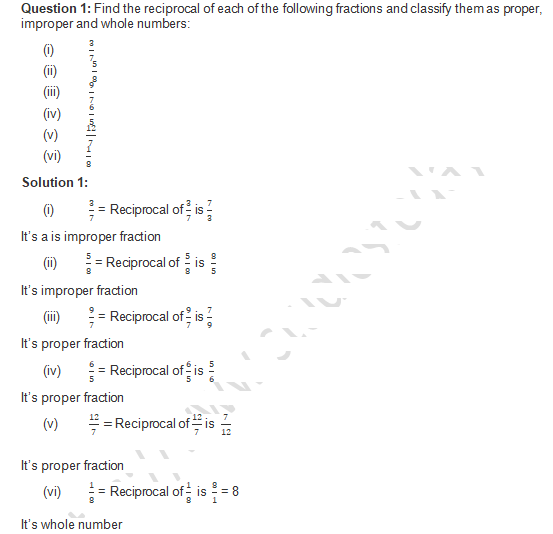Question 2:  Divide:
(i)  3/8   by  5/9
(ii) 3 1/4 by  2/3
(iii)   7/8   by 4 1/2
(iv) 6 1/4   by 2 3/5

Solution 2:

(i) 3/8   by  5/9
According to the rule of division fraction ( a/b) ÷ (c/d) = (a/b) × (d/c)
3/8   ÷  5/9 =   3/8   ×  9/5
= (3 ×9 )/(8 × 5)
=27/40

(ii) 3 1/4 by  2/3
Covert mixed fraction into improper fraction
= 3 1/4 =( 4 ×3+1) =    13/4
According to the rule of division fraction ( a/b) ÷ (c/d) = (a/b) × (d/c)
13/4   ÷  2/3 =   13/4   ×  3/2
= (13 × 3 )/(3 × 2)
=  26/6
= 4 7/8

(iii) 7/8   by 4 1/2
Covert mixed fraction into improper fraction
= 4 7/8 =( 2 ×4+1) =   9/2
According to the rule of division fraction ( a/b) ÷ (c/d) = (a/b) × (d/c)
7/8   ÷  9/2 =   7/8   ×  2/9
= (7 × 2 )/(8 × 9)
= 14/72
=  7/36

(iv) 6 1/4   by 2 3/5
Covert mixed fraction into improper fraction
= 6 1/4   =( 4 ×6+1) =    25/4
= 2 3/5      =( 5 ×2+3) =   13/5
According to the rule of division fraction ( a/b) ÷ (c/d) = (a/b) × (d/c)
25/4   ÷  13/5 =   25/4   ×  5/13
= (25 × 5 )/(4 × 13)
= 125/52
=  2 21/52

Question 3. Divide:
(i)  3/8      by 4
(ii) 9/16     by 6
(iii) 9 by  3/16
(iv) 10 by  100/3

Solution 3:

(i) 3/8      by 4
=3/8     ÷ 4
= (3/8     × 4)
=   3/32

(ii) 9/16     by 6
= 9/16     ÷ 6
= (9/16 × 6)
=  9/96
= 3/32

(iii) 9 by  3/16
= 9 ÷ (3/16)
= ((9 × 16)/3)
= 16 × 3
= 48

(iv) 10 by  100/3
= 10 ÷  100/3
= ((10 × 3)/100)
= (3/10)

Question 4. Simplify:
(i) 3/10  ÷ 10/3
(ii) 4 3/5÷   4/5
(iii) 5 4/7 ÷ 1  3/10
(iv) 4 ÷ 2  2/5

Solution 4:

(i)  3/10  ÷ 10/3
= (33 × 3)/(10 × 10)
=  9/100

(ii) 4 (3 )/5   ÷   4/5
Covert mixed fraction into improper fraction
= 4 (3 )/5   =( 5 ×4+3) =    23/5
= 23/5  ÷ 4/5
= (23 ×5)/(5 × 4)
=  23/4 = 53/4

(iii) 5 4/7 ÷ 1  3/10
Covert mixed fraction into improper fraction
= 5 4/7   =( 7 ×5+4) =    39/7
=1  3/10   =( 10 ×1+3) =    13/10
= 39/7  ÷ 13/10
= (39 ×10)/(7 × 13)
=  390/91 =   30/7  = 5( 2)/7

(iv) 4 ÷ 2  2/5
Covert mixed fraction into improper fraction
= 2  2/5   =( 5 ×2+2) =    12/5
= 12/5  ÷ 4
= (4 ×5)/12
=  20/12 = 1  2/3

Question 5. A wire of length 12 1/2 m is cut into 10 pieces of equal length. Find the length of each piece.

Solution 5:

Total length of the wire = 12 1/2
Covert mixed fraction into improper fraction
= 12  1/2   =( 2 ×12+1) =    25/2 m.
Wire is cut into 10 pieces, so length of one piece is
= 25/2 ÷10
= 25/20
=   5/4  = 1 1/4 m
The length of 1 piece is 1 1/4 m.

Question 6. The length of rectangular plot of area 65 1/3 m2 is 12 1/4 m. What is the width of the plot?

Solution 6:

Area of rectangular plot is 65 1/3  m2
Length of the same plot is 12 1/
Covert mixed fraction into improper fraction
= 65 1/3 =( 3 ×65+1) =    196/3 m2

= 12  1/4   =( 2 ×12+1) =    49/4 m.
Area of rectangle = length × breadth
= (196  ×49)/(3 × 4)
=  784/147 = 5  1/3
The width of the plot is 5  1/3.

Question 7. By what number should 6 2/9 be multiplied to get 4 4/9?

Solution 7:

Let x be the number which needs to be multiplied by 6 2/9
Covert mixed fraction into improper fraction
= 6 2/9  =(  9 ×6+2) =    56/9
= 4 4/9 = (  9 ×4+4) =    40/9
x × (56 )/9  = 40/9
x =  40/9×   9/56
x = 40/56
x = 5/7
The number is 5/7.

Question 8 The product of two numbers is 25 5/6. If one of the numbers is 6 2/3., find the other.

Solution 8:

Product of two numbers is 25 5/6
One of the numbers is 6 2/3
Covert mixed fraction into improper fraction
= 25 5/6 =(  6 ×25+5) =    155/6
= 6 2/3 = (  3 ×6+2) =    20/3
Let x the other number
155/6  = x × 20/3
x = 3/20  ×  155/6
x =  31/8
x = 3  7/8
The other number is 3  7/8

Question 9: The cost of 6 (1 )/(4 )  kg of apples is Rs 400. At what rate per kg are the apples being sold?

Solution 9:

The cost of 6 (1 )/(4 ) kg = ((25 )/(4 )) of apples is Rs 400.
Cost of apple per kg is = ((25 )/(4 )) /400
= ((4 )/(25 )) × 400
= Rs. 64
Therefore, the cost of apples per kg. are 64 Rs.

Question 10: By selling oranges at the rate of Rs 5 (1 )/(4 )  per orange, a fruit-seller gets Rs 630. How many dozens of oranges does he sell?

Solution 10:

The cost of 1 orange is Rs 5  (1 )/(4 )   = (21/4]
Mixed fraction covert into fraction;
5  (1 )/(4 )=(4 × 5 + 1) = (21 )/(4 )
By selling the oranges he got Rs. 630
Number of dozens of oranges sold by him for Rs 630 is = ((4 )/(21 )) × 630
= 120 apples
As we know that 1 dozen = 12
120 apples means 10 dozens.

Question 11: In mid-day meal scheme (3 )/10 liter of milk is given to each student of a primary school. If 30 liters of milk is distributed every day in the school, how many students are there in the school?

Solution 11:

Milk is given to each student = (3 )/10 liter
No. of student given  (3 )/10 liter of milk = 1
No. of students giving 1 liter of milk = (10 )/3
No. of students giving 30 liters of milk = (10 )/3 × 30 = 100 students
Therefore, 100 students are there in school.

Question 12: In a charity show Rs 6496 were collected by selling some tickets. If the price of each ticket was Rs 50 (3 )/4, how many tickets were sold?

Solution12:

Amount collected by selling tickets is Rs. 6496
The Amount per ticket is = 50 (3 )/4=   203/4
Number of tickets bought at Rs.  203/4 = 1
Number of tickets bought by Rs 6496 s = 4/203 (4/203) × 6496
= 4 × 32
= 128 ticket